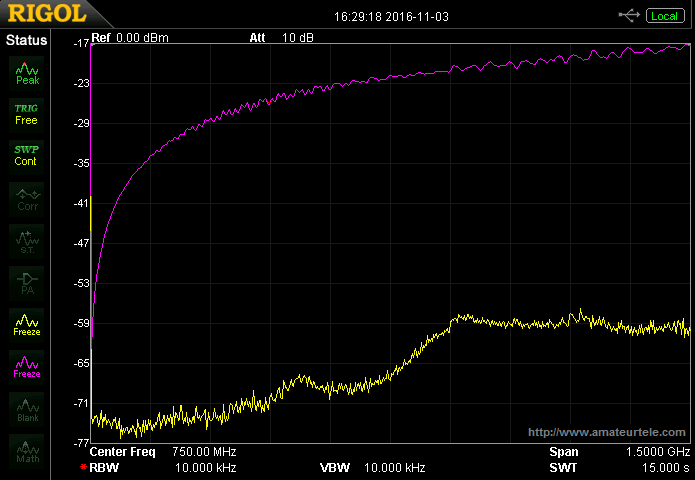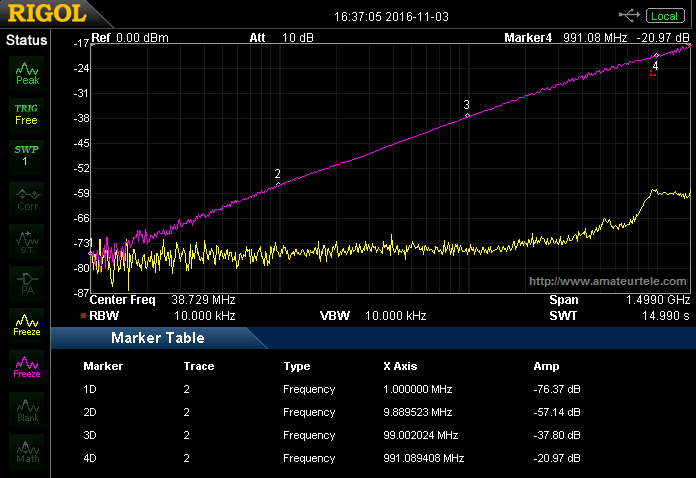A long time ago I bought a Radio System AB (Ericsson) 50 Ohms dummy load with N-connector for testing purposes. It’s a dummy load from the former NMT450 mobile phone network. It’s likely these dummy load were fitted to the front of the RS460 cavity band pass filter. The footprint, paint color and structure and four holes of the dummy load match perfectly to the unpainted square on the front of the RS460 filter boxes. Possibly the dummy load were used to dissipate sideband energy…The nice thing about these dummy loads it equipped with a BNC test point. The internal chip resistor has a test point which is connected to the BNC socket. This is for example convenient for frequency measurements. The RF power is dissipated into the chip resistor and a relative low power test signal can be used to feed a frequency counter. I guess the chip resistor can handle at least 100 Watts of RF power when fitted to a heatsink.The dummy load is used for seven years now. And since I bought a Rigol DSA815-TG spectrum analyzer recently, this is a nice opportunity to determine the power reduction at the test point. I normalized the test setup and measured the loss. I would expect the signal reduction is linear, but I was wrong. The signal reduction of the test point is logarithmic. The lower the frequency, the more the signal reduction at the test point and vice versa. Luckily I updated the firmware of the Rigol DSA815-TG spectrum analyzer and has now the option to show the frequency scale logarithmic instead of linear. Therefore the bent signal reduction line on the screen appears quite linear! Using the CSV export function I exported the trace information to Microsoft Excel. After fiddling with the graph function I added a “trend” line that fits very nice onto the trace of the measurement. There’s a convenient option to show the formula of the trend line. Since the trend line fits very well it’s safe to say that the formula fits therefore also the signal reduction. The determined formula is this: [dB] = (7,527 × f [MHz])-72,44. If a frequency in MHz is multiplied with 7,527 and the result is reduced with 72,44, the answer is the signal reduction in dB relative to the input.For convenience I calculated the signal reduction for the regular ham bands. The results are shown below. The dummy load is used for approximately 460 MHz and should work fine up to 3 GHz. I cannot verify this since my equipment can measure “only” op to 1,5 GHz. Assuming the formula fits until 10 GHz, the numbers should fit. But it’s possible the dummy load will behave different at “extreme” frequencies (above 3 GHz). So beware not to blow up the input of your measurement devices. Normally a spectrum analyzer is very sensitive for overload at the input. Be sure to calculate/verify the test point signal strength at certain frequencies and input power. For example the Rigol DSA815-TG can handle +20dBm at max. If a HF transmitter (1,8…28 MHz) is tested, the least signal reduction at the test point is -47,4 dB. The maximum signal at the input port if the dummy load is +67,4 dBm. The maximum input power is therefore 5.495 Watts. Since the dummy load can handle approximately 100 Watts, it’s safe to say that your DSA815 won’t be damaged testing an 100 Watts HF transmitter. At 435 MHz the signal reduction is 26,7 dB. The maximum input is +20 dBm, therefore the maximum power input is +20 dBm + 26,7 dB = +46,7 dBm = 46,8 W! There are several 70 cm transceivers and amplifiers delivering more than 50 Watts, so beware that this could (or will?!) damage the DSA815 applying more than 46,8 Watts to the dummy load input.1,8 MHz = -68,0 dB3,5 MHz = -63,0 dB5,3 MHz = -59,9 dB7 MHz = -57,8 dB10 MHz = -55,1 dB14 MHz = -53,6 dB18 MHz = -50,7 dB21 MHz = -49,5 dB25 MHz = -48,2 dB28 MHz = -47,4 dB50 MHz = -43,0 dB145 MHz = -35,0 dB435 MHz = -26,7 dB1,3 GHz = -18,472,3 GHz = -14,2 dB*3,4 GHz = -11,2 dB *5,8 GHz = -7,2 dB *10,0 GHz = -3,1 dB ** These numbers are based on the formula and not verified in a real world measurement.
 inside